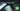# Hackerrank | Solution of Breaking the Records in Golang

October 2nd, 2018
|

In this one we will solve question breaking the records from hackerrank in golang. Let's dive right into it.

# Problem Statement

The question can be found at this link. The problem statement states that we are given a list of scores and we need to find out how many times the records of lowest and highest scores were broken.

# Challenges

• Choose our preferred language as golang on hackerrank. The moment we do that, we get some 50-60 lines of code which are very unfamiliar to someone who is new to language.
• Cracking the logic required to solve the question.

# Solution

This is the template you get on hackerrank for this problem statement.

``````    package main

import (
"bufio"
"fmt"
"io"
"os"
"strconv"
"strings"
)

// Complete the breakingRecords function below.
func breakingRecords(scores []int32) []int32 {

}

func main() {

stdout, err := os.Create(os.Getenv("OUTPUT_PATH"))
checkError(err)

defer stdout.Close()

writer := bufio.NewWriterSize(stdout, 1024 * 1024)

checkError(err)
n := int32(nTemp)

var scores []int32

for i := 0; i < int(n); i++ {
scoresItemTemp, err := strconv.ParseInt(scoresTemp[i], 10, 64)
checkError(err)
scoresItem := int32(scoresItemTemp)
scores = append(scores, scoresItem)
}

result := breakingRecords(scores)

for i, resultItem := range result {
fmt.Fprintf(writer, "%d", resultItem)

if i != len(result) - 1 {
fmt.Fprintf(writer, " ")
}
}

fmt.Fprintf(writer, "\n")

writer.Flush()
}

if err == io.EOF {
return ""
}

return strings.TrimRight(string(str), "\r\n")
}

func checkError(err error) {
if err != nil {
panic(err)
}
}``````
• First go ahead and delete everything in the pre-existing template.
• Let's write down our main wrapper function.
``````package main
import "fmt"

func main(){

}``````

Let's think about the variables we would need. We need the number of scores `n`, the nax score `max`, min score `min`, maximum counter `cmax`, minimum counter `cmin`. Now do we need to take the array as an input or we can just store each element in temporary variables and be done with it. We don't need to store array elements for any future reference, so we would need only one temporary variable `t`. Let's declare these variables and take input.

``````
...
var n,min,max,cmin,cmax,t int
fmt.Scan(&n)``````

Now we need to loop over `n` times and take scores as input in our temprary variables. Let's do that and then we will decide our logic.

``````	...
for i:=0;i<n;i++{
fmt.Scan(&t)
//logic here
}``````

Let's discuss the logic. For the first score, whatever the score is, that is the lowest and highest for the games, after that whatever we get we need to check it with the lowest and highest number to see if it broke the record. If yes, we increase the corresponding counter and update the min/max variable with new one. Let's implement this.

``````	...
for i:=0;i<n;i++{
fmt.Scan(&t)
if i ==0 {
min = t
max = t
}else{
if t < min {
cmin++
min = t
}
if t > max{
max = t
cmax++
}
}
}
fmt.Println(cmax,cmin)``````

Okay, we have the for loop that we had earlier, we take our score, if the index is `0` i.e. first score, we assign min and max variable to the the score. If the index is something else, we check two conditions. First, if the score is lower than the previous minimum, we update the minimum and increrase the counter, second, if the score is more than the previous maximum, we update the max variable and increase the counter. Next after the loop, we print the counters in the required formats. Now your complete code should look like

``````package main
import "fmt"

func main(){
var n,min,max,cmin,cmax,t int
fmt.Scan(&n)

for i:=0;i<n;i++{
fmt.Scan(&t)
if i ==0 {
min = t
max = t
}else{
if t < min {
cmin++
min = t
}
if t > max{
max = t
cmax++
}
}
}
fmt.Println(cmax,cmin)

}``````

This is it for this one, complete source code for this post can be found on my Github Repo. Will see you in the next one.